# Introduction

This package determines species probabilities (i.e., relative abundances) that satisfy a given functional trait profile. Restoring resilient ecosystems requires a flexible framework for selecting assemblages that are based on the functional traits of species. However, current trait-based models have been limited to algorithms that can only select species by optimising specific trait values, and could not elegantly accommodate the common desire among restoration ecologists to produce functionally diverse assemblages. We have solved this problem by applying a non-linear optimisation algorithm that optimises Rao Q, a closed-form functional trait diversity index that incorporates species abundances, subject to other linear constraints. This framework generalises previous models that only optimised the entropy of the community, and can optimise both functional diversity and entropy simultaneously. This package can also be used to generate experimental assemblages to test the effects of community-level traits on community dynamics and ecosystem function.

There are two functions in this package:
selectSpecies( ): This function generates the species assemblages according to the user-defined trait profile.
plotProbs( ): This function plots the relative abundances of the species along the axes of either one or two traits.

## The selectSpecies( ) function takes the following arguments:

t2c: Traits to constrain: A matrix of species trait values, where species are organized as rows and traits as columns. These should be numeric arrays, and no missing values are tolerated. Ordinal data will yield meaningful results, but nominal data entered as numeric vectors are invalid. Row and column names are optional. This matrix can be any dimension, but the number of traits should be less than the number of species.

constraints: Trait constraints: A vector of trait values that serve as constants in the constraint equations. If not specified, the function will not constrain solutions to trait means. These trait contraints must be listed in the same order as columns in t2c. These constraints are community-weighted mean trait values, i.e., average traits weighted by the relative abundance of each species.

t2d: Traits to diversify: Can be 1) a matrix of species trait values to diversify where species are organized as rows and traits as columns. NAs are tolerated as long as each pair of species can be compared by at least one trait. In this case, dissimilarities among species are computed using Euclidean distance. The number of species in t2d must match those in t2c. Or 2) a distance matrix of class dist that contains dissimilarities among species, no NAs are tolerated in the distance matrix.

obj: the objective function that is maximized. It can be one of three possibilities (QH, Q, H). QH = Quadratic entropy (Q) plus entropy (H); Q = Quadratic entropy; H = entropy.

phi: A parameter bounded between 0 and 1 that weights the importance of either quadratic entropy or entropy (default = 0.5). Phi of 1 corresponds to 100 percent Q, phi of 0.5 corresponds to 50 percent Q and 50 perfect H, phi of 0 corresponds to 100 percent H.

capd: A logical stating whether the distance matrix should be capped at the mean distance among species (default = FALSE). Mean distance is calculated as the average of all upper triangular entries in the distance matrix calculated from t2d.

euclid: A logical stating whether the distance matrix should be transformed to an Euclidean matrix if necessary (default = TRUE).

## The plotProbs() function takes the following arguments:

result: A saved object from function selectSpecies()

traits: A matrix of trait values where traits are columns and rows are species. If one trait is provided, then the function creates a 2D barplot of probabilities for each species. If two traits are provided, then the function creates a 3D barplot that illustrates probabilities of species located within a 2D trait space.

colors: An optional vector of colors for plotting that must include at least two valid color names. The default color scheme is a ramp palette of lightblue to blue.

xlim: Vector of two numbers denoting limits on x-axis.

ylim: Vector of two numbers denoting limits on y-axis.

xlab: Character string to describe x-axis.

ylab: Character string to describe y-axis.

zlab: Character string to describe z-axis (default = Probabilities).

distance: An optional number denoting distance between bars in 3d plot.

cex.lab: An optional number denoting the size of the labels. The default is set to 1.5.

box.col: An optional setting for the color of the box. The default setting is transparent.

xbase: The length of the base of each 3d bar along the x-axis

ybase: The length of the base of each 3d bar along the y-axis

# Part 1. Examples using one trait with known structure (evenly spaced integers)

Create a simple trait dataset for examples 1 through 4 using a species pool of 5 species with trait values 1 through 5.

### Set size of species pool
Spp <- 5

### Set trait values for each species as integers from 1 to 5 (Spp)
### Note that we make this a matrix to pass it into the function
trait <- as.matrix(data.frame(trait = c(1:Spp)))

### Most datasets have species names as row names, so let's add arbitrary row names using letters
rownames(trait) <- c(letters[1:nrow(trait)])

## Example 1: Derive species assemblage with the following trait profile: {CWM = 3.5}

Let us start with the most basic use of the function: to derive a species abundance distribution where we only want to constrain the abundances so that the community has a community-weighted mean (CWM) trait equal to 3.5. This is equivalent to applying the FD::maxent function.

We will define four arguments in the function for this example t2c: this is the matrix of species trait values that we want to constrain constraints: this is a vector of CWM trait values. In this case, we only have a one dimensional matrix of t2c, so this vector should contain only one element: 3.5 t2d: when you are not maximizing functional diversity, simply specify the same matrix here as you did for t2c obj: this is the objective function that is being maximized. In this example we are not maximizing functional diversity, so we use the entropy function (H)

### Store results from selectSpecies() into 'result1'
result1 <- selectSpecies(t2c = trait, constraints = c(trait=3.5), t2d = trait, obj = "H")
#>
#> Iter: 1 fn: -1.5461   Pars:  0.11205 0.14490 0.18738 0.24231 0.31335
#> Iter: 2 fn: -1.5461   Pars:  0.11205 0.14490 0.18738 0.24231 0.31335
#> solnp--> Completed in 2 iterations

# Use the plotProbs() function to plot the abundance distribution
plotProbs(result1, trait, xlab = "Species")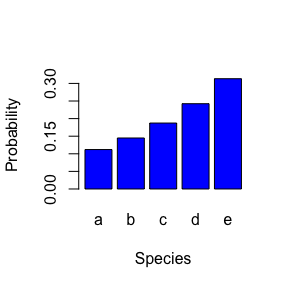Note that these results are equivalent to the FD::maxent function because all we are doing is constraining the species abundance distribution to have a CWM of 3.5 while maximizing entropy.

### compare result1 with identical maxent output from FD package. Be sure to have the FD package installed.
round(FD::maxent(constr = c(3.5), states = trait)$prob, 5) #> a b c d e #> 0.11206 0.14490 0.18738 0.24231 0.31335 round(t(result1$prob), 5)
#>            a      b       c       d       e
#> [1,] 0.11205 0.1449 0.18738 0.24231 0.31335

## Example 2: Derive species assemblage with the following trait profile: {CWM = 3.5, maximize Rao Q}

We will now see what happens when we maximize Rao Q (Q, quadratic entropy), an index of functional diversity, rather than maximizing entropy.

### Store results from selectSpecies() into 'result2'. Note the only difference with result1 is a different objective function (Q).
result2 <- selectSpecies(t2c = trait, constraints = c(trait=3.5), t2d = trait, obj = "Q")
#>
#> Iter: 1 fn: -0.9375   Pars:  0.37494261 0.00004128 0.00003222 0.00004128 0.62494261
#> Iter: 2 fn: -0.9375   Pars:  0.374990526 0.000006815 0.000005319 0.000006815 0.624990526
#> solnp--> Completed in 2 iterations

# plot result2
plotProbs(result2, trait, xlab = "Species")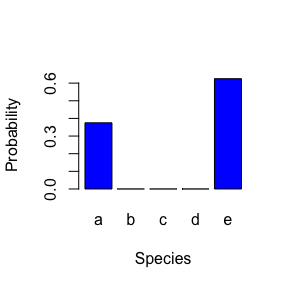Interestingly, the CWM trait value is the same for both result1 and result2, but the species abundance distributions are radically different. When maximizing Rao Q, this makes the most functionally dissimilar species the most abundant, and all species in the middle of the trait distribution have vanishingly small abundances. This is not a desirable solution for ecological restoration. One way to fix this is to optimize both Rao Q and Entropy simultaneously.

## Example 3: Derive species assemblage with the following trait profile: {CWM = 3.5, maximize Rao Q and Entropy}

We will now see what happens when we maximize a function that additively combines both Rao Q (Q, quadratic entropy) and entropy (H).

### Store results from selectSpecies() into 'result3'. Note the only difference with result2 is a different objective function (QH)
result3 <- selectSpecies(t2c = trait, constraints = c(trait=3.5), t2d = trait, obj="QH")
#>
#> Iter: 1 fn: -1.1690   Pars:  0.16129 0.13048 0.13704 0.18931 0.38188
#> Iter: 2 fn: -1.1690   Pars:  0.16129 0.13048 0.13704 0.18931 0.38188
#> solnp--> Completed in 2 iterations

# plot result3
plotProbs(result3, trait, xlab = "Species")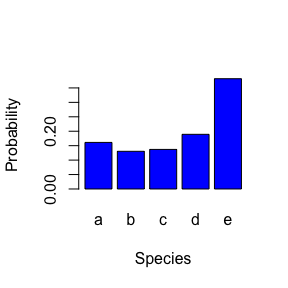Note that the abundance distribution still maximizes the most dissimilar species, but it evens out the abundances across all the species.

## Example 4: Derive species assemblage with the following trait profile: {maximize Rao Q and Entropy, no CWM trait constraint}

Suppose we do not want to constrain the abundances to satisfy a specific CWM trait value, and simply want to maximize functional diversity. If you do not want to constrain the results to satisfy a particular CWM trait value, then leave the t2c and constraints arguments blank.

### Store results from selectSpecies() into 'result4'
result4 <- selectSpecies(t2d = trait, obj = "QH")
#>
#> Iter: 1 fn: -1.2267   Pars:  0.26398 0.16465 0.14275 0.16465 0.26398
#> Iter: 2 fn: -1.2267   Pars:  0.26398 0.16465 0.14275 0.16465 0.26398
#> solnp--> Completed in 2 iterations

# plot result4
plotProbs(result4, trait, xlab = "Species")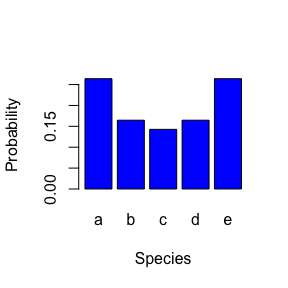# Part 2. Examples using two traits with known structure (evenly spaced integers)

In many cases, we will want to restore ecological communities with convergence toward one trait value, but we want to diversify a different trait. The following examples illustrate how to do so on a dataset with known structure to easily illustrate the results.

Create 2-dimensional trait matrix

### 2-dimensional trait matrix of 16 species, evenly spaced between trait values 1 through 4
trait.matrix <- as.matrix(cbind(traitX = c(rep(1,4), rep(2,4), rep(3,4), rep(4,4)), traitY = c(rep(c(1,2,3,4),4))))

### specify species names
rownames(trait.matrix) <- c(letters[1:16])

### Example 5: Derive species assemblage with the following trait profile: {constrain trait X to a CWM = 3.5, maximize Rao Q of trait Y}

### Create two evenly-spaced orthogonal traits
traitX <- matrix(c(rep(1,4), rep(2,4), rep(3,4), rep(4,4)))
traitY <- matrix(c(rep(c(1,2,3,4),4)))
rownames(traitX) <- c(letters[1:16]); colnames(traitX) <- c("traitX")
rownames(traitY) <- c(letters[1:16]); colnames(traitY) <- c("traitY")

### Store results from selectSpecies() into 'result5'
result5 <- selectSpecies(t2c = traitX, constraints = c(traitX=3.5), t2d = traitY, obj = "Q", capd = FALSE)
#>
#> Iter: 1 fn: -0.7500   Pars:  0.00754771 0.00001153 0.00001155 0.00783403 0.05296666 0.00001153 0.00001155 0.05287494 0.12106497 0.00001152 0.00001155 0.12096817 0.31837454 0.00001153 0.00001155 0.31827669
#> Iter: 2 fn: -0.7500   Pars:  0.007548054 0.000004746 0.000004777 0.007836191 0.052978252 0.000004745 0.000004773 0.052885955 0.121079576 0.000004747 0.000004775 0.120982151 0.318375110 0.000004746 0.000004775 0.318276628
#> solnp--> Completed in 2 iterations

### plot result5
trait.matrix <- cbind(traitX, traitY)
plotProbs(result5, trait.matrix, cex.lab = 0.7)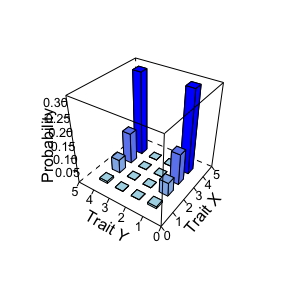Note how this result is not desirable because it suppresses the abundances of species with intermediate trait Y values. This is because we only maximized Rao Q. Let us see how the results change when we maximize both Rao Q and entropy.

### Example 6: Derive species assemblage with the following trait profile: {constrain trait X to a CWM = 3.5, maximize Rao Q + Entropy of trait Y}

### Store results from selectSpecies() into 'result6'
# obj: select the QH objective function to maximize both Rao's Q and H, and cap the distance matrix by setting capd = TRUE
result6 <- selectSpecies(t2c = traitX, constraints = c(traitX=3.5), t2d = traitY, obj = "QH", capd = TRUE)
#>
#> Iter: 1 fn: -1.3823   Pars:  0.008029 0.007527 0.007527 0.008029 0.022088 0.020708 0.020708 0.022089 0.060768 0.056970 0.056970 0.060768 0.167178 0.156732 0.156732 0.167178
#> Iter: 2 fn: -1.3823   Pars:  0.008029 0.007527 0.007527 0.008029 0.022088 0.020708 0.020708 0.022089 0.060768 0.056970 0.056970 0.060768 0.167178 0.156732 0.156732 0.167178
#> solnp--> Completed in 2 iterations

### plot result6
plotProbs(result6, trait.matrix, cex.lab = 0.7)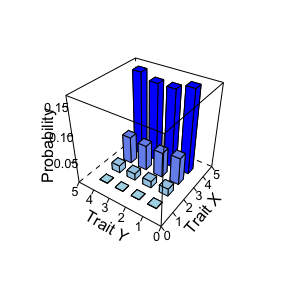Note how this result evens out the abundances of species across the trait Y axis, while still creating a community with an average trait X value of 3.

# Part 3. An example using a real dataset

## Example 7: Derive species assemblage for a California serpentine grassland with the following trait profile: {constrain water use efficiency to a CWM = 67th percentile, maximize Rao Q of rooting depth}

Restoring ecosystems that are resilient to drought is often an important management goal. Drought tolerant plants can exhibit high water use efficiency (WUE), the rate of carbon assimilation per unit of water used. Therefore, selecting species with traits that converge on high water use efficiency is one goal of the project. Rooting depth also influences drought tolerance, but a drought-resilient community would likely exhibit a diversity of rooting depths to optimize complementary water use throughout the soil profile. Therefore, selecting species that optimize rooting depth diversity would be important.

Using a dataset of 48 species from a serpentine grassland in California, we first log transform and then standardize each variable to unit variance.

### load serpentine data (available in the R package)
serpentine <- read.csv("traits.serpentine.california.csv", header = TRUE, row.names = 1)

### take logs and rescale to unit variance
wue <- data.frame(wue = scale(log(serpentine$wue))) rootdepth <- data.frame(rootdepth = scale(log(serpentine$rootdepth)))

### transfer species row names
rownames(wue) <- rownames(serpentine)
rownames(rootdepth) <- rownames(serpentine)

# set constraint on wue as the 67% quantile of wue distribution
wue.constraint <- c(quantile(wue\$wue, 0.67))
names(wue.constraint) <- c("wue")

We use the selectSpecies function to derive an assemblage with a high average WUE by constraining the assemblage to the 67th percentile of the distribution of WUE, but diversified the range of rooting depths by optimizing QH with a capped distance matrix. This output can be used to design a seed or planting mix for a restoration project by selecting species with the highest probabilities.

### Store results from selectSpecies() into 'result7'
result7 <- selectSpecies(t2c = as.matrix(wue), constraints = c(wue.constraint), t2d = as.matrix(rootdepth), capd = TRUE, obj = "QH")
#>
#> Iter: 1 fn: -2.0531   Pars:  0.023419 0.020731 0.011602 0.009973 0.004511 0.002142 0.012328 0.019188 0.014306 0.035379 0.034306 0.016926 0.013409 0.020047 0.018127 0.018052 0.033891 0.024428 0.032579 0.024375 0.023935 0.009173 0.007774 0.026075 0.011090 0.007744 0.022727 0.022472 0.016667 0.007751 0.016469 0.025725 0.024250 0.045120 0.027970 0.033529 0.012026 0.011684 0.009747 0.007439 0.016363 0.044099 0.018705 0.052368 0.014732 0.016531 0.049228 0.028893
#> Iter: 2 fn: -2.0531   Pars:  0.023418 0.020730 0.011601 0.009972 0.004512 0.002142 0.012327 0.019188 0.014307 0.035380 0.034308 0.016927 0.013409 0.020047 0.018127 0.018052 0.033892 0.024427 0.032578 0.024375 0.023933 0.009174 0.007777 0.026074 0.011088 0.007742 0.022725 0.022472 0.016668 0.007749 0.016470 0.025724 0.024249 0.045121 0.027970 0.033528 0.012027 0.011682 0.009745 0.007441 0.016364 0.044100 0.018705 0.052367 0.014733 0.016532 0.049228 0.028893
#> solnp--> Completed in 2 iterations

### plot result7
plotProbs(result7, traits = cbind(wue,rootdepth), xlab = "Water use efficiency", ylab = "Rooting depth", colors = c("darkolivegreen4", "gold2"), cex.lab = 0.7)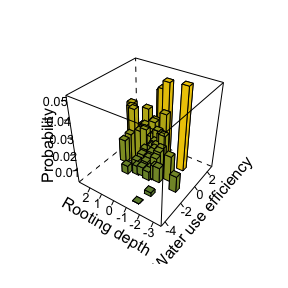Note that this figure illustrates how the species with the highest probabilities exhibit a range of rooting depths but generally high water use efficiency.